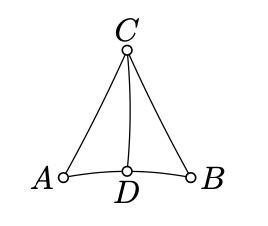$$\newcommand{\id}{\mathrm{id}}$$ $$\newcommand{\Span}{\mathrm{span}}$$ $$\newcommand{\kernel}{\mathrm{null}\,}$$ $$\newcommand{\range}{\mathrm{range}\,}$$ $$\newcommand{\RealPart}{\mathrm{Re}}$$ $$\newcommand{\ImaginaryPart}{\mathrm{Im}}$$ $$\newcommand{\Argument}{\mathrm{Arg}}$$ $$\newcommand{\norm}{\| #1 \|}$$ $$\newcommand{\inner}{\langle #1, #2 \rangle}$$ $$\newcommand{\Span}{\mathrm{span}}$$

# 11.4: Defect

$$\newcommand{\vecs}{\overset { \rightharpoonup} {\mathbf{#1}} }$$ $$\newcommand{\vecd}{\overset{-\!-\!\rightharpoonup}{\vphantom{a}\smash {#1}}}$$$$\newcommand{\id}{\mathrm{id}}$$ $$\newcommand{\Span}{\mathrm{span}}$$ $$\newcommand{\kernel}{\mathrm{null}\,}$$ $$\newcommand{\range}{\mathrm{range}\,}$$ $$\newcommand{\RealPart}{\mathrm{Re}}$$ $$\newcommand{\ImaginaryPart}{\mathrm{Im}}$$ $$\newcommand{\Argument}{\mathrm{Arg}}$$ $$\newcommand{\norm}{\| #1 \|}$$ $$\newcommand{\inner}{\langle #1, #2 \rangle}$$ $$\newcommand{\Span}{\mathrm{span}}$$ $$\newcommand{\id}{\mathrm{id}}$$ $$\newcommand{\Span}{\mathrm{span}}$$ $$\newcommand{\kernel}{\mathrm{null}\,}$$ $$\newcommand{\range}{\mathrm{range}\,}$$ $$\newcommand{\RealPart}{\mathrm{Re}}$$ $$\newcommand{\ImaginaryPart}{\mathrm{Im}}$$ $$\newcommand{\Argument}{\mathrm{Arg}}$$ $$\newcommand{\norm}{\| #1 \|}$$ $$\newcommand{\inner}{\langle #1, #2 \rangle}$$ $$\newcommand{\Span}{\mathrm{span}}$$

The defect of triangle $$\triangle ABC$$ is defined as

$$\text{defect} (\triangle ABC) := \pi - |\measuredangle ABC| - |\measuredangle BCA| - |\measuredangle CAB|.$$

Note that Theorem 11.3.1 states that the defect of any triangle in a neutral plane has to be nonnegative. According to Theorem 7.4.1, any triangle in the Euclidean plane has zero defect.

Exercise $$\PageIndex{1}$$

Let $$\triangle ABC$$ be a nondegenerate triangle in the neutral plane. Assume $$D$$ lies between $$A$$ and $$B$$. Show that

$$\text{defect} (\triangle ABC) = \text{defect} (\triangle ADC) + \text{defect} (\triangle DBC).$$Hint

Note that $$|\measuredangle ADC| + |\measuredangle CDB| = \pi$$. Then apply the definition of the defect.

Exercise $$\PageIndex{2}$$

Let $$ABC$$ be a nondegenerate triangle in the neutral plane. Suppose $$X$$ is the reflection of $$C$$ across the midpoint $$M$$ of $$[AB]$$. Show that

$$\text{defect} (\triangle ABC) = \text{defect} (\triangle AXC).$$Hint

Show that $$\triangle AMX \cong \triangle BMC$$. Apply Exercise $$\PageIndex{1}$$ to $$\triangle ABC$$ and $$\triangle AXC$$.

Exercise $$\PageIndex{3}$$

Suppose that $$ABCD$$ is a rectangle in a neutral plane; that is, $$ABCD$$ is a quadrangle with all right angles. Show that $$AB = CD$$.

HintShow that $$B$$ and $$D$$ lie on the opposite sides of $$(AC)$$. Conclude that

$$\text{defect} (\triangle ABC) + \text{defect} (\triangle CDA) = 0.$$

Apply Theorem $$\PageIndex{1}$$ to show that

$$\text{defect} (\triangle ABC) = \text{defect} (\triangle CDA = 0$$

Use it to show that $$\meauredangle CAB = \measuredangle ACD$$ and $$\measuredangle ACB = \measuredangle CAD$$. By ASA, $$\triangle ABC \cong \triangle CDA$$, and, in particular, $$AB =CD$$.

(Alternatively, you may apply Exercise 11.3.1)

Advanced Exercise $$\PageIndex{4}$$

Show that if a neutral plane has a rectangle, then all its triangles have zero defect.